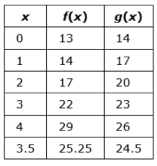# Sample Test Item PreviewExport Print
General Information
Related Benchmark: MAFS.912.A-REI.4.11
Reporting Category: Algebra: Reasoning with Equations & Inequalities
Type: EE: Equation Editor
Difficulty: N/A
Question:

Cora is using successive approximations to estimate a positive solution to f(x)=g(x), where

f(x)=x²+13 and g(x)=3x+14. The table shows her results for different input values of x.Use Cora's process to find the positive solution, to the nearest tenth, of f(x)=g(x).